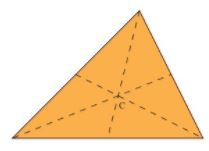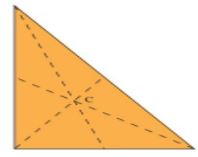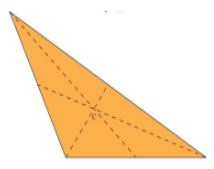# Theory - To illustrate that the Medians of a Triangle Concur at a Point (called the Centroid), Math Class 9 Notes | EduRev

## Class 9 : Theory - To illustrate that the Medians of a Triangle Concur at a Point (called the Centroid), Math Class 9 Notes | EduRev

The document Theory - To illustrate that the Medians of a Triangle Concur at a Point (called the Centroid), Math Class 9 Notes | EduRev is a part of the Class 9 Course Mathematics (Maths) Class 9.
All you need of Class 9 at this link: Class 9

Objective:

To illustrate that the medians of a triangle concur at a point (called the centroid), which always lies inside the triangle.

Theory:

A centroid of a triangle is the point where the three medians of the triangle meet. A median of a triangle is a line segment from one vertex to the mid-point on the opposite side of the triangle.

The centroid is also called the center of gravity of the triangle. If you have a triangle plate, try to balance the plate on your finger. Once you have found the point where it will balance, that is the centroid of that triangle.

Properties of the centroid:

• It is always located inside the triangle.

• The centroid divides each median in a ratio of 2:1. In other words, the centroid will always be 2/3 of the way along any given median.

• In below images, c is centroid for triangles(a, b & c).Acute-angled(a)Right-angled(b)Obtuse-angled(c)

Offer running on EduRev: Apply code STAYHOME200 to get INR 200 off on our premium plan EduRev Infinity!

## Mathematics (Maths) Class 9

190 videos|233 docs|82 tests

,

,

,

,

,

,

,

,

,

,

,

,

,

,

,

,

,

,

,

,

,

,

,

,

;